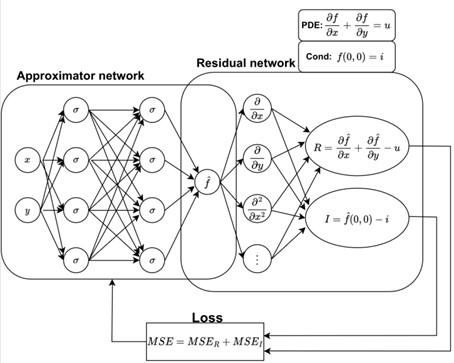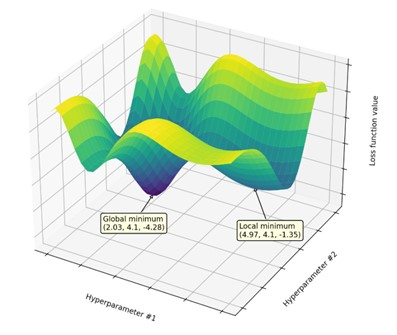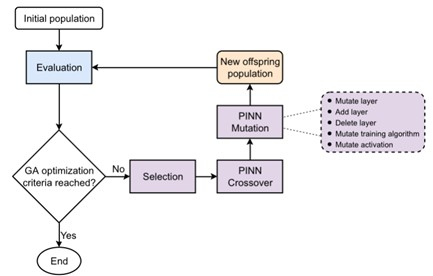### Optimization of Physics Informed Neural Networks (PINN)

•Milos Ivanovic
• Aug 28, 2023

Artificial intelligence is a field that is developing at a dizzying speed, but do these new algorithms really "understand" the problems they practically solve? In this article, we will deal with Physics Informed Neural Networks (PINN), which provide a powerful tool for integrating real physical laws into a neural network. We will also learn how the Blackfox tool intelligently optimizes their architecture and other hyper-parameters.

#### Machine learning and science

Traditionally, any scientific research is a cyclical combination of a theoretical proposition and an experimental verification. In the last few decades, computer simulations, which are also based on established theoretical laws, are often used in addition to real experiments. In physics, engineering, biology, economics and other fields, laws are mostly represented by partial differential equations. For example, the well-known logistic equation, the ordinary differential equation of the first order represents the population growth model:

$$\frac{df}{dt} = R \cdot t(1-t)$$

The function f(t) gives the population growth rate over time t, while the parameter R determines the maximum growth rate. In order to choose a single curve as a solution from the family of curves that satisfy the solution of this ordinary differential equation, we must set the so-called boundary or (in this case) initial condition. Let it be:

$$f(t=0) = \frac{1}{2}.$$

The solution of the equation with the given boundary condition is the function shown in the following figure:

We can easily solve this ordinary differential equation analytically, as well as with some numerical method, such as the finite difference method. On the other hand, the machine learning approach is completely different. Models such as neural networks, decision trees and Support Vector Machines are mainly driven by the existence of large amounts of data. Let's say, the curve in the above picture could be faithfully reproduced (and thus predict the solution of our logistic equation) if we have available several hundred points belonging to it. Such a model would probably produce solid results, but without any knowledge of the logistic law behind it.

#### Disadvantages of a data-only approach

If we tried to determine the value of the function for some point outside the domain used for training, the results would probably be poor. Second, if there is noise in the data, the question of how much such irregularity would mislead a model based on the data. This is the well-known overfitting and underfitting problem.A classic machine learning approach that is purely data-driven. Great opportunity to either overfit or underfit. On the other hand, a huge number of phenomena have been explained and described by partial differential equations. Why don't we use the equation we know in order to "fit" the curve as correctly as possible and prevent overfit? The following figure illustrates three realistic data availability scenarios:

Most of today's data-only models belong to the Big Data type (far right), while classical approaches such as the finite difference method or the finite element method belong to the Lots of physics type (far left). The innovative PINN method aims to reconcile these two extreme approaches. In the real world, we always know something about the physical nature of the problem, and we also have some measured data. Let's say, maybe we don't know the exact value of the constant R in our logistic equation, but we have measured values of the population f(t) at several time points t. It's a very realistic scenario.

#### PINN – Physics Informed Neural Network

Physics Informed Neural Network is a machine learning technique that can be used to approximate the solution of a partial differential equation.Architecture of PINN and standard training loop constructed for solving a simple partial differential equation

PDE and Cond denote the equations and boundary conditions of the problem at hand, while R and I represent their residuals, which should tend to zero. An approximator network is a "regular" neural network that undergoes a training process and provides an approximate solution f ̂. The key to the method is in the so-called residual network. It is a special part of PINN that is not trained, and is capable of calculating the derivatives of the output of the approximator network with respect to the inputs, resulting in a combined loss function, denoted by MSE.

#### Why a model that gives 0 for any input?

Rules in the form of differential equations and boundary conditions are actually integrated into the residual network. If the given rules are satisfied, the PINN outputs R and I are ZERO! Since the rules are set to return zero only if the function f ̂ given by the approximation network is correct, then we have a training mechanism that will iteratively converge to that exact solution.

The training data is actually not real data as in supervised learning. In the so-called collocation points within the problem domain we calculate the MSE loss, tending to minimize them during the training process. In other words, we are searching for such values of the weighting coefficients so that the differential equation and the boundary conditions are satisfied in all collocation points.

Automatic differentiation is a key driver of PINN development and is the main element that distinguishes PINN from similar efforts in the 1990s. Today, we rely on automatic differentiation implemented in deep learning frameworks such as Tensorflow and PyTorch. In the past few years, several libraries have appeared that make working with PINNs much easier, so their users can focus on the problem they are solving, and leave the technical details to the library itself. The most famous libraries of this type are SciANN and the increasingly popular DeepXDE.

Various problems of interest in practice can be solved with the help of PINNs. Specific problems and solutions in the field of heat conduction, oscillations, acoustics, hydrology and solar energy can be found in the Web edition of the PINN Praktikum (in Serbian Language) published by the Faculty of Science and Mathematics in Kragujevac, signed by the author of this article. Solutions in the form of Python code can be downloaded from https://github.com/imilos/pinn-skripta. The author is also happy to accept corrections and suggestions of any kind, preferably pull requests.

#### Which PINN architecture is best?

As with any classification/regression model in the field of machine learning, the question arises which PINN hyper-parameters achieve the best results? Hyper-parameters primarily include the architecture expressed by the number of layers and the number of neurons in each layer, activation functions, training algorithm, etc. This is not a simple task at all, because the search space is generally huge and the computing resources are limited. In addition, no one guarantees that, for example, more complex networks will give more accurate results, as illustrated in the figure.Model hyper-parameter optimization problem. We try to find the combination that gives the global minimum, where the model is most accurate.

Let's say if training of one single network takes 10 minutes, it takes us 16 hours to test 100 hyper-parameter combinations, which is usually not nearly enough to find a PINN close to optimal.

#### Method 1: Random search

Simple grid search or related random search can be easily run by utilizing the SciKit Learn functions. If we have a multi-core processor, we can significantly speed up the search through parallelization.

However, is there any better solution than grid and random search?

#### A smarter search for optimal architecture

In the absence of a better solution, today even serious research is carried out using the humoristically entitled Graduate Student Descent, ie. completely manually. Let's see if we can find a optimization algorithm that can help us in our search.

#### Method 2: Hyperopt TPE

The proven method Hyperopt offers hyper-parameter optimization for a variety of machine learning models (not just neural networks). The authors use the advanced Tree-structured Parzen Estimator (TPE) technique, which during the search dynamically determines its further course, so that those branches of the tree where better models are more likely to appear are examined. Specific details can be found in the original paper. Here is an example of how we can define the hyper-parameter search space, as a preparation for Hyperopt TPE run:

    MAX_LAYERS = 10
MIN_UNITS = 16
MAX_UNITS = 32

# Next, define a search space for Hyperopt.
search_space = {
'activation' : hp.choice('activation',  ["tanh", "sigmoid", "selu", "softmax", "relu", "elu"]),
'outputActivation': hp.choice('outputActivation',  ["linear", "relu"]),
}
layers_choice = []
for layer_count in range(1, MAX_LAYERS + 1):
options = []
for i in range(1, layer_count + 1):
units =  hp.quniform(f'layers_{layer_count}_unit_{i}', MIN_UNITS, MAX_UNITS, 0.9)
options.append(units)
layers_choice.append(options)
search_space['layers'] =  hp.choice('layers', layers_choice)

We have 4 types of search algorithms, 6 activation functions, 2 output layer activations, which is not too many combinations. However, what makes this search really challenging are all the combinations of PINN topologies. There can be a maximum of 10 layers, and each layer can have an arbitrary number of neurons (UNITS) from 16 to 32!

#### Method 3: Blackfox Search Based on Genetic Algorithm (PINN/GA)

The research group of the Center for Computer Modeling and Optimization (CERAMO) at Faculty of Science, University of Kragujevac in cooperation with the Vodéna company has been dealing with optimization methods of various machine learning models for several years. One of the results is the Blackfox project, which is used in various practical domains, such as the optimization of production and consumption of electricity from renewable sources, the telecom industry and the optimization of supply chains in the retail industry.

Let's see how Blackfox's built-in algorithms deal with PINNs. Blackfox should find the optimal PINN for the given problem completely automatically, with its genetic algorithm specialized for searching the spaces that make up neural networks. Blackfox PINN/GA starts from a population of very simple PINN networks, and adds new layers to them only if such a move results in a clear gain in accuracy. In this way, we should also fulfill the secondary goal, to make the optimal PINN as simple as possible. Simplicity brings a benefit in performance, and does not come at the expense of quality. The flow of the optimization process is given in the following figure.Genetic algorithm loop for optimal PINN architecture search. Each individual represents different PINN network

In a genetic algorithm, the population of PINNs evolves toward better solutions. Each PINN has a set of traits (genes) that can be mutated, ie. changed. Evolution starts from a population of randomly generated simplest PINNs. In each new generation, the accuracy of each PINN is evaluated separately. The better the PINN scores, the more likely it survives and crossover with one of the other PINNs in the population. What is the speciality of Blackfox is the change (mutation) which can be:

1. Changing the number of neurons in the hidden layer
2. Inserting a new hidden layer
3. Deleting a hidden layer
4. Selection of a long training algorithm
5. Selection of another activation function

In order to be able to evaluate as many PINNs as possible per a unit of time, Blackfox has the ability to utilize a Kubernetes cluster, i.e. to distribute the load transparently on several computers and thereby speed up the optimization procedure multiple times.

#### Benchmark setup

Which of the three methods will find the best PINN for a specific problem? Let's compare the performance of all three methods on a representative problem. We choose Stefan's ice melting problem in one spatial dimension, described in detail in the PINN Praktikum (in Serbian), complete with equations, boundary conditions, and Python code. Here we will only show a diagram of what is modeled.

We model the motion of the boundary between water and ice s(t) and the temperature field u(x,t) in water.

To test all three optimization methods to their limits of usability, we use a cluster consisting of 7 servers with 32 processor cores each (dual Intel Xeon E5-2683 v4), giving us a total of 224 processors that can function in parallel. Random Search and Blackfox distribute work using Kubernetes, while Hyperopt TPE uses Apache Spark for parallelization. Since they all run on the same hardware, the results are comparable. Further details of the testing can be found in our paper published in Applied Soft Computing journal.

#### Results

How much does each PINN optimization method improve over time (120 minutes)? The left Y-axis shows the error of the model, and the right Y axis shows the complexity of the PINN network in the number of trainable parameters.

The solid lines represent the error of the current best PINN (lower the better), while the dashed lines represent the complexity of the current best PINN (lower is better).

At the very beginning, a random search (red line) finds a very good, but quite complex network, better than Hipopert TPE. However, systematic improvement over time is lacking because the algorithm is based on chance. Hyperopt TPE (green line) shows a good gradient at the beginning, steadily improves and after 120 minutes finishes slightly better than random search. On the other hand, Blackfox (PINN/GA) (blue line) gradually and systematically improves by adding new layers and remains better than both competing methods almost all the time, while keeping PINNs fairly simple. It reaches a steady state after about 120 minutes. Due to the specific implementation of the mutation operator, we set all PINNs' initial populations to exactly one hidden layer. As Blackfox PINN/GA progresses, we can watch the models become more and more complex. Sometimes the complexity is even reduced as a result of the layer removal operator, which acts as a kind of regularization mechanism.

At the very end, here are the optimal architectures and hyper-parameters that each method found after 2 hours of searching. Blackfox PINN/GA found a network that with only two hidden layers matches the network with as many as 7 hidden layers provided by Hyperopt TPE.

#### Conclusion

The optimized Blackfox PINN/GA model provides demonstrably better accuracy than the PINN obtained by parallel random search as well as the Hyperopt TPE method, in the same distributed computing environment. In most cases, Blackfox keeps the network topology relatively simple. From the user's point of view, for the sake of better performance, it is worth waiting a few hours to get the optimal combination of hyper-parameters.